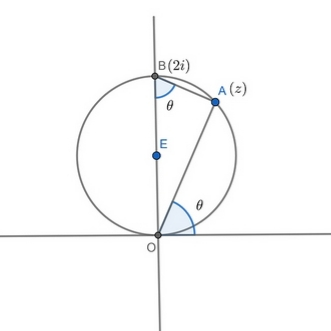# Doubt from complex numbers

I got |z| = 2 sin^2 (theta) now how to proceed from here..? Ans is i. My expression is not simplifying. .

If you are interested in doing this question fastly.
We proceed by z=1+i.
First Condition satistied.
You get thita as tan^-(1)=45.
Substitute
1-(2/1+i)
1-21-i/1+i1-i
1-2(1-i)/2
1-1+i=i

3 Likes

dnt have pen paper now i m sorry (travelling).

1 Like
3 Likes

|z-i|=1 represents a circle centered at i and radius 1 as depicted above

Note that \angle OAB = 90^{\circ} (since OB is a diameter)

Also \angle OBA = 90^{\circ} - \angle BOA = \theta

We have \cot \theta =\left| \dfrac{z-2i}{z}\right| and \arg \left(\dfrac{z-2i}{z} \right) = -\dfrac{\pi}{2}

Hence \dfrac{z-2i}{z} = 1 - \dfrac{2i}{z} = -i \cot \theta

Multiplying by i throughout we get i + \dfrac{2}{z}= \cot \theta

or \cot \theta - \dfrac{2}{z}=i

7 Likes

Although Harishankar Bhatt sir already solved that problem.

Using Bhatt Sir Diagram.....We can write z=2\sin(\theta)\cdot e^{i\theta}.

\displaystyle \Longrightarrow \cot(\theta)-\frac{2}{z}=\frac{\cos \theta}{\sin\theta}-\frac{(\cos \theta-i\sin\theta)}{\sin \theta}=i.

6 Likes

Oh that was much shorter!

Thanks @Jagdish_Singh

2 Likes